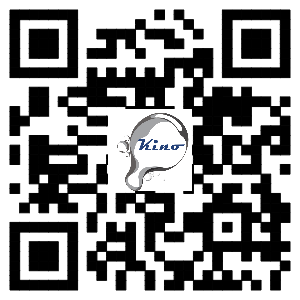﻿ PPT for how to calibration and verification of the contact angle meter
Welcome to the KINO: The world leading supplier of contact angle meter, surface & interfacial tensiometer &TOF camera
##### Position
PPT for how to calibration and verification of the contact angle meter
2019-2-19 17:04:58

PPT for how to calibration and verification of the contact angle meter.

According to the development and application time, the verification tools of the contact angle meter are mainly divided into three types :(1) microscope reticle, (2) standard contact angle plate of circle or Young-Laplace shape and (3) professional 3D tools for verification the contact Angle meter.

üMany test standard from ISO, ASTM or GB (Chinese Standard) are used for Contact angle meter as shown below. But almost all of them used WH method which uses in older contact angle goniometer. And almost all of them is the standard for how to use contact angle goniometer for its application. So, test standard now cannot be used to calibrate contact angle meter.
üContact angle meter used WH was used for 100 years and it is now is falling behind due to it is just a geometry value and has no repeatability and just . Modern contact angle meter uses Young-Laplace equation fitting method or ADSA-P which is conform to surface chemistry principle.

•What contact angle characterizes? The parameters for calculating contact angle？Verification or calibration?

Contact angle meter is the instrument for measuring contact angle between solid-liquid-air (or fluid), and it can also be used to measure surface tension (L/G) or interface tension (L/F).  It characterizes physicochemical property of solid. So, we will also use water contact angle meter to name the contact angle meter which uses water as the probe liquid to describe the hydrophilic and hydrophobic surface of solid and the cleanness of solid surface.

Surface tension, contact angle are the two property index from contact angle meter. The measurement standard categories involved in these two indicators are: contact angle is at category 8 - geometric measurement: also known as length measurement, which is the measurement of the geometric size, position and Angle of the object; surface tension is category 10 - stoichiometry: also known as physical stoichiometry, it refers to the analysis and measurement of the composition, physical properties and basic physical constants of various substances.

So, we have two method for verifying the contact angle meter：
1, Contact angle: Measuring geometric parameters of a standard object (3D) and measuring contact angle of the same 3D object.
Then, verifying the difference between them to finish the verification of contact angle meter. The difference value of them
could be changed to a acceptable value by leveling the sample stage and view angle of lens.
2, Surface tension: Measuring surface tension of liquid with known value of surface tension and verifying the difference of
them. The verification of contact angle meter is almost same as viscometer but we cannot calibrate the value of surface
tension.

Verifying the contact angle meter, not calibrating it, because we will not and cannot adjust the parameter to meet the know value.

##### Focus news